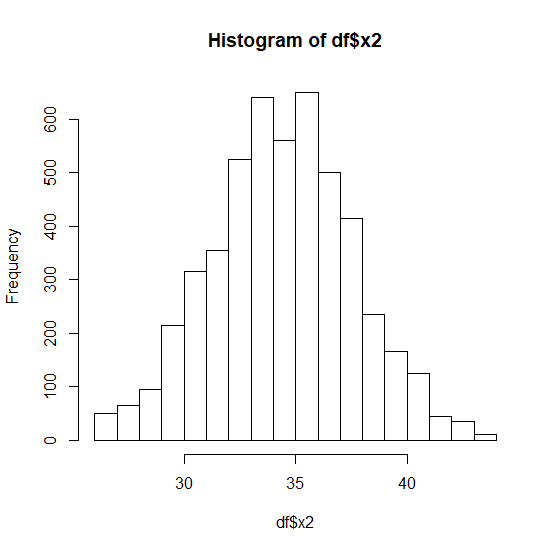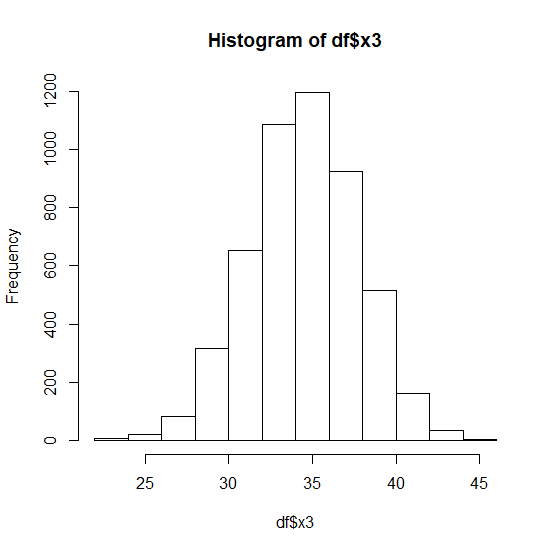# How to visualize the normality of a column of an R data frame?

The first step to analyze a variable is checking whether it is normally distributed or not and to visually do this, we create a histogram. If the histogram depicts a bell then we consider that the variable is normally distributed otherwise, it is not. We can create a histogram for any column of an R data frame by using hist function.

## Example

Consider the below data frame −

set.seed(9)
df<-data.frame(x1=rbinom(100,50,0.7),x2=rbinom(1000,50,0.7),x3=rbinom(5000,50,0.7))

Creating the histograms for x1, x2, and x3 −

## Outputhist(df\$x3)

## Output